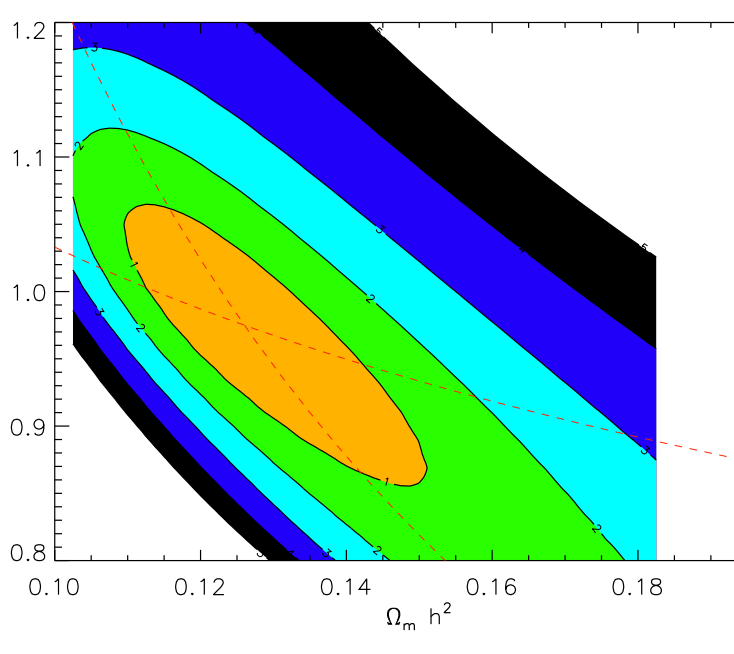Detecting Baryon Acoustic OscillationsAuthors: A. Labatie, J.-L. Starck, M. Lachièze-Rey Journal: ApJ Year: 2012 Download: ADS | arXiv

Abstract

Baryon Acoustic Oscillations are a feature imprinted in the galaxy distribution by acoustic waves traveling in the plasma of the early universe. Their detection at the expected scale in large-scale structures strongly supports current cosmological models with a nearly linear evolution from redshift approximately 1000, and the existence of dark energy. Besides, BAOs provide a standard ruler for studying cosmic expansion. In this paper we focus on methods for BAO detection using the correlation function measurement. For each method, we want to understand the tested hypothesis (the hypothesis H0 to be rejected) and the underlying assumptions. We first present wavelet methods which are mildly model-dependent and mostly sensitive to the BAO feature. Then we turn to fully model-dependent methods. We present the most often used method based on the chi^2 statistic, but we find it has limitations. In general the assumptions of the chi^2 method are not verified, and it only gives a rough estimate of the significance. The estimate can become very wrong when considering more realistic hypotheses, where the covariance matrix of the measurement depends on cosmological parameters. Instead we propose to use a new method based on two modifications: we modify the procedure for computing the significance and make it rigorous, and we modify the statistic to obtain better results in the case of varying covariance matrix. We verify with simulations that correct significances are different from the ones obtained using the classical chi^2 procedure. We also test a simple example of varying covariance matrix. In this case we find that our modified statistic outperforms the classical chi^2 statistic when both significances are correctly computed. Finally we find that taking into account variations of the covariance matrix can change both BAO detection levels and cosmological parameter constraints.

Summary

We have designed a specific wavelet adapted to search for shells, and exploit the physics of the process by making use of two different mass tracers, introducing a specific statistic to detect the BAO features. We have applied our method to the detection of BAO in a galaxy sample drawn from the Sloan Digital Sky Survey (SDSS). We have used the "main" catalogue to trace the shells, and the luminous red galaxies (LRG) as tracers of the high density central regions. Using this new method, we detect, with a high significance, that the LRG in our sample are preferentially located close to the centers of shell-like structures in the density field, with characteristics similar to those expected from BAO (Arnalte-Mur, Labatie, Clerc, Martínez,  Starck et al, A&A, 2012). Then we have studied the classical method for detecting BAOs and the assumptions that the method requires. We have also found that the approximation of a constant covariance matrix in the classical BAO analysis method can affect non negligibly both the BAO detection and cosmological parameter constraints (Labatie, Starck, Lachieze-Rey, ApJ,2012a) (Labatie, Starck, Lachieze-Rey, ApJ,2012b).